•  LaTeX 科技排版：包含水平三线图式的伪代码算法排版algorithm    http://blog.renren.com/share/66049300/679318404   中文环境下使用 http://wwv.renren.com/xn.do?ss=10791&rt=1 ...

﻿﻿

LaTeX 科技排版：包含水平三线图式的伪代码算法排版algorithm

下面这个文档对排版算法至关重要，请多加练习

http://blog.renren.com/share/66049300/679318404    中文环境下使用  http://wwv.renren.com/xn.do?ss=10791&rt=1 http://www.sciencenet.cn/m/user_content.aspx?id=231910  Latex live 指南 Latex 符号指南     Latex 公式指南  Latex 图像指南  http://www.latex-project.org/intro.html  http://latex.yo2.cn/articles/latex-learning0.html  2008/8/3 Latex 使用心得  最近学会了使用 Latex ，并且今年的 First Year Report 就是用 Latex 写的，发现了很多也许有用的小技巧，总结一下。
工具：
我现在使用的是 CTex ，一个号称支持中文的 Latex ，不过我现在还用不上中文。该软件免费可下载：
http://www.ctex.org/HomePage   里面的 WinEdit 确实是很好用的。编译的内核是 MikTex 2.4 ，有一点老，不过基本功能都有了。
在 linux 上，可以使用 texmaker ，用了用，还是不错的。
一些使用心得 :
插入图片。在 Latex 中，图片是以文件的方式嵌入到文档当中，在转换为 pdf 或者 ps 文件的时候才会嵌入到文件中，否则都是单独存在的。插入图片的基本命令：
\begin{figure}
\centering
\includegraphics[width=0.6\textwidth]{file/vcrouter}
\caption{Internal structure of a VC router}\label{fig:vcrouter}
\end{figure}
\begin{figure} 和 \end{figure} 中间是图片的命令。 \centering 之后的所有内容居中。 \includegraphics 插入图片， width=0.6\textwidth 说明图片的宽度为 0.6 倍页宽，文件名是 file/vcrouter ，用 latex 编译自动搜索后缀为 eps 的图像， pdflatex 编译自动搜索后缀为 pdf 的文件。 \caption 说明该图 片的标题， \label 给出一个标签，文中则可以使用 \ref{} 进行连接。插入图片需要加载 \usepackage{graphicx} 。
插入多幅图片并包含子图的图片：
\begin{figure}[ht]
\centering \subfigure[A bundled-data channel]{
\includegraphics[width=0.30\textwidth]{file/bundleddata}\label{fig:bundleddata}}
\hspace{0.1\textwidth} \subfigure[The 4-phase bundled-data
protocol]{
\includegraphics[width=0.4\textwidth]{file/4phasebundled}\label{fig:4phasebundled}}
\caption{The 4-phase bundled-data protocol}\label{fig:4pb}
\end{figure}
这是一个两个子图水平并列的例子。在 \begin{figure} 后添加 [ht] 说明以水平 table 的形式排布，当然也可以使用 tabular ，不过麻烦一些。使用 \includegraphics 需要加载 \usepackage{subfigure} 。
公式编辑。其实可以使用公式编辑器。 MathType 5.0 以上，在 perferences 菜单里的 translators 选择 translate to other languages ，然后选择 latex 。之后，用公式编辑器编辑的公司可以直接用选择和复制放到 latex 文件当中。
如果公式需要加编号，使用 \begin{equation} 和 \end{equation} 就能自动添加编号。不过最好加载 \usepackage{amsmath} 。另外，默认公式是居中的，如果需要改成靠左缩进的方式，在 \documentclass[fleqn] {firstyearreport} 添加这个 fleqn 选择参数。
参考文献最好使用 bibtex 管理。管理的软件可以使用 Endnote ，不过我用的是 jabref ，一个开源软件，还是很好用的。只要 写上 \bibliography{file/reference} ，这里的 file/reference 说明参考文献是 file /reference.bib 文件，所有的参考文献就可以自动加载。关于参考文献的风格，我使用的 是 \bibliographystyle{alpha} ，以作者的第一字母和年代为标号。但是还有很多其他的方式，可以参考这个网站： http://www.cs.stir.ac.uk/~kjt/software/latex/showbst.html
图片格式是一个很烦人的问题。最基本的图片格式是 eps ，尽管现在 pdflatex 支持 pdf 和 jpeg,png 等等，但是我还是认为 eps 比较好。 eps 是矢量图，没有图像失真。用 eps 转换成的 pdf 放大多少都没有问题，体积也很小。
但是，支持 eps 的软件并不多。在 windows 上，我们最习惯的是 Visio 画图，但是 visio 对 eps 基本上没有支持。网上有很多将 visio 的图转化成 eps 的图的方法，但是很多都很麻烦。我现在终于找到了一种比较好的方式。
首先安装一个 postscript 的虚拟打印机， http://www.adobe.com/support/downloads/detail.jsp?ftpID=1502 。然后用 visio 将图片用 postscript 打成 prn 或者 ps 文件。用 CTex 自带的 GSview 打开该文件（没有也没关系，下一个： http://pages.cs.wisc.edu/~ghost/ ）， file 菜单中有一个 ps to eps ，哈哈，自动转换边界，就变成 eps 文件了，而且是矢量的。
还有一个问题， pdflatex 偏偏是不支持 eps 文件，默认是 pdf 文件。使用 pdflatex 时，如果没有 pdf 文件会报错。有人说使 用 \usepackage{epstopdf} 可以解决该问题， eps 文件会自动在编译时变为 pdf 文件，但是在 windows 上的使用结果很糟 糕， eps 文件没有自动转换边界，按 A4 打印，结果很难看。
其实加载 \usepackage{epstopdf} ，就是使用 epstopdf 命令转换 eps 文件。但是在 windows 系统中的 epstopdf 命令好像不能自动转换边界，但是 linux 系统上的 epstopdf 是好的。所以我建议使用 linux 系统上的 epstopdf 命令转 化，是会自动转化边界的。
不过大批的文件一个一个去手动转化还是很麻烦，我就写了一个 makefile 文件，假设所有的 eps 文件都在一个文件夹下，那么 make all 一下，就能自动转化为 pdf 文件。知道我在说什么吧，呵呵。 Makefile 的内容如下：
clean:
rm -f *.pdf
eps_file = $(wildcard *.eps) pdf_file =$(eps_file:%.eps=%.pdf)
$(pdf_file): %.pdf : %.eps epstopdf$<
all: $(pdf_file) show: echo$(pdf_file)
伪代码。伪代码有时候还是要用的，对于复杂的算法，直接写伪代码有时候更容易懂。关于伪代码有一个包 algorithms ，需要加 载
\usepackage{algorithm}和
\usepackage{algorithmic} ， 具体用法可以直接看他的帮助，在下载的压缩包中的 doc 目录下。下载路径：
http://www.ctan.org/tex-archive/help/Catalogue/entries/algorithms.html   忘了说了，所有 Latex 相关的文件找不到，或者需要最新版，请查询
http://www.ctan.org/tex-archive/help/Catalogue/entries/algorithms.html   .

伪代码举例 ：

\usepackage{algorithm}    %format of the algorithm  \usepackage{algorithmic}  %format of the algorithm

\begin{algorithm}
\caption{Calculate $y = x^n$}
\label{alg1}
\begin{algorithmic}
\REQUIRE $n \geq 0 \vee x \neq 0$
\ENSURE $y = x^n$
\STATE $y \leftarrow 1$
\IF{$n < 0$}
\STATE $X \leftarrow 1 / x$
\STATE $N \leftarrow -n$
\ELSE
\STATE $X \leftarrow x$
\STATE $N \leftarrow n$
\ENDIF
\WHILE{$N \neq 0$}
\IF{$N$ is even}
\STATE $X \leftarrow X \times X$
\STATE $N \leftarrow N / 2$
\ELSE[$N$ is odd]
\STATE $y \leftarrow y \times X$
\STATE $N \leftarrow N - 1$
\ENDIF
\ENDWHILE
\end{algorithmic}
\end{algorithm}

效果 ：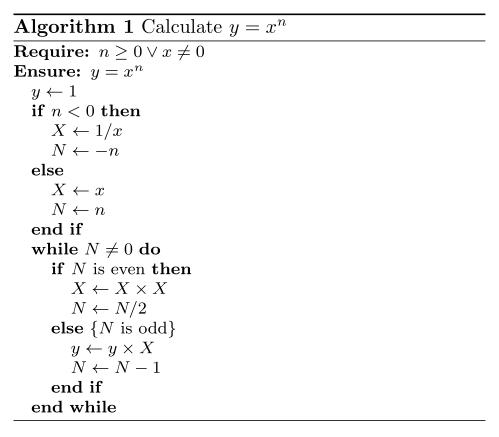algorithm宏包的文档指导 ：
/Files/Lhw978/algorithms.pdf

LaTex：算法排版
http://www.binghe.org/2010/03/typeset-algorithm-in-latex/
排版可能需要的包：
usepackage{algorithm}    %format of the algorithm
usepackage{algorithmic}  %format of the algorithm
usepackage{multirow}     %multirow for format of table
usepackage{amsmath}
usepackage{xcolor}
DeclareMathOperator*{argmin}{argmin}                   %argmin或argmax公式的排版
enewcommand{algorithmicrequire}{ extbf{Input:}}   %Use Input in the format of Algorithm
enewcommand{algorithmicensure}{ extbf{Output:}} %UseOutput in the format of Algorithm

排版图片可能需要的包：
usepackage{graphics}
usepackage{graphicx}
usepackage{epsfig}

算法的排版举例( 例子代码有误) ：
\begin{algorithm}[htb] %算法的开始
caption{ Framework of ensemble learning for our system.} %算法的标题
label{alg:Framwork} %给算法一个标签，这样方便在文中对算法的引用
\begin{algorithmic} %这个1 表示每一行都显示数字
REQUIRE ~~\
%算法的输入参数：Input
The set of positive samples for current batch, $P_n$;\
The set of unlabelled samples for current batch, $U_n$;\
Ensemble of classifiers on former batches, $E_{n-1}$;
ENSURE ~~\
%算法的输出：Output
Ensemble of classifiers on the current batch, $E_n$;
STATE Extracting the set of reliable negative and/or positive samples $T_n$ from $U_n$ with help of $P_n$; label{code:fram:extract} %算法的一个陈述，对应算法的一个步骤或公式之类的； label{ code:fram:extract }对此行的标记，方便在文中引用算法的某个步骤
STATE Training ensemble of classifiers $E$ on $T_n cup P_n$, with help of data in former batches; label{code:fram:trainbase}
STATE $E_n=E_{n-1}cup E$; label{code:fram:add}
STATE Classifying samples in $U_n-T_n$ by $E_n$; label{code:fram:classify}
STATE Deleting some weak classifiers in $E_n$ so as to keep the capacity of $E_n$; label{code:fram:select}
RETURN $E_n$;
%算法的返回值
end{algorithmic}
end{algorithm}

排版效果图 ：在文中对算法和算法的某个步骤的引用：Therefore, in step
ef{code:fram:extract} of algorithm
ef{alg:Framwork}, we extract $T_n$, a set of reliable negative samples
1、 For和While循环语句的排版举例
（1） 排版效果图（2）排版代码(
例子代码有误)
\begin{algorithm}[h]
caption{An example for format For & While Loop in Algorithm}
\begin{algorithmic}
FOR{each $iin [1,9]$}
STATE initialize a tree $T_{i}$ with only a leaf (the root);\
STATE $T=T?igcup T_{i};$\
ENDFOR

FORALL {$c$ such that $cin RecentMBatch(E_{n-1})$} label{code:TrainBase:getc}
STATE $T=T cup PosSample(c)$; label{code:TrainBase:pos}
ENDFOR;

FOR{$i=1$; $i<n$; $i++$ }
STATE $//$ Your source here;
ENDFOR

FOR{$i=1$ to $n$}
STATE $//$ Your source here;
ENDFOR

STATE $//$ Reusing recent base classifiers. label{code:recentStart}
WHILE {$(|E_n| leq L_1 )and( D eq phi)$}
STATE Selecting the most recent classifier $c_i$ from $D$;
STATE $D=D-c_i$;
STATE $E_n=E_n+c_i$;
ENDWHILE label{code:recentEnd}

end{algorithmic}
end{algorithm}

算法 algorithm 重命名

使用 \usepackage{algorithm2e} 如何 使得 caption {}排版结果产生的“Agorithm”变为 " 算法 "？

milksea 回复：
改这两个
\renewcommand{\listalgorithmcfname}{List of Algorithms}
\renewcommand{\algorithmcfname}{Algorithm}

问题解决

，谢谢

附录。需要插入附录的话，下面的命令会很有用
\appendix
\appendixpage
\appendix 说明之后的内容为附录， \appendixpage 将添加一个专门的附录页， \addappheadtotoc 将附录添 加到目录当中，需要加载 \usepackage{appendix} 。不过，一旦附录开始，将不能转回正文。另一种方式可以使用 \begin{appendices} 和 \end{appendices} 在正文中添加附录，参看 http://www.tex.ac.uk/cgi-bin/texfaq2html?label=appendix
关于文档中的引用链接和生成 pdf 的链接目录，只能使用 pdflatex 。方法是加载 \usepackage{hyperref} ，所有链接自动生成。
关于所有的 latex 相关的命令，有一本手册（书）
http://tobi.oetiker.ch/lshort/lshort.pdf ，好像有中文的翻译版本 http://net.ytu.edu.cn/share/%D7%CA%C1%CF/lshort-cn.pdf
哈哈，还有一个事， space 访问量过万了。 2008 年 8 月 3 日 。

源地址：
http://www.cnblogs.com/Lhw97 8/admin/javascript:showReg(0);                                           
展开全文• word2016中写出伪代码
最近写硕士论文，发现有些个算法还是需要贴一些伪代码进去，那么如何在word里写格式优雅的伪代码呢？ 上网查过结论就是，利用名叫Aurora的word插件来完成。
1 安装
下载Aurora安装包：云盘链接，密码t1s6。

你可以去Aurora的官网下载。反正我是打不开，加VPN也打不开，据说软件到2009年就不更新了，可能官网也挂了吧。此安装包来自网络，是带有注册机的破解版。

如果电脑之前没有miktex，建议安装最新版miktex2.9。从 官网 下载并双击安装。并记住安装位置；关闭office。双击Aurora安装包，不要勾选安装miktex的选项，直到安装完成；

不建议勾选安装过程中的miktex选项，是因为如果勾选了这个选项的话，在Aurora自己安装完成后会试图联网下载miktex2.5并安装。我亲测过，这样会联网失败下载不下来，并且据说2.5版本不太支持。此外，2.8版本也不支持，所以，会是徒劳操作。

将电脑系统时间调到2009年某天，双击下载的破解机，输入任意英文字母，破解成功。

调整系统时间后会不能正常上网，windows可能也会显示未激活，如果你的word版本像我（2016）一样在2009年之后的话。不要在意这些，没什么大问题。

打开word。如果是32位的word，会在选项卡中出现Aurora选项。听说之前如果是64位word，则不会出现选项卡，只能通过插入——对象——Aurora Equation来插入latex代码。不过我现在测试是可以的，可能新版office对其添加了支持吧。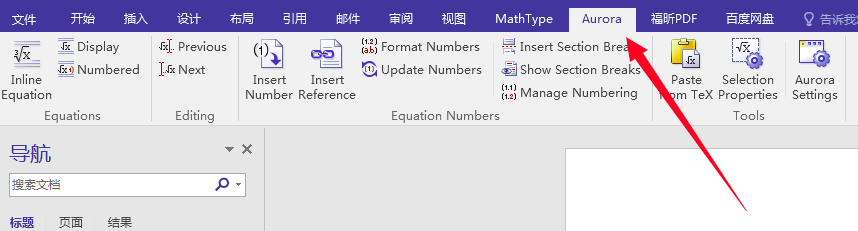点击插入方程式（Inline Equation）会出现一个窗口，在窗口中可进行latex代码编辑。首先，点击properties——paths，将安装的miktex中latex，dvipng和pdflatex的路径填写在对应的位置。修改完成后点击应用。路径通过刚才记住的安装位置查找，如果实在找不到，可搜索miktex文件夹。以下是我的安装路径（默认，无修改）可供参考。latex,dvipng 和pdflatex都在此文件夹下 D:\MiKTeX\miktex\bin\x64

在properties选项卡，将Rendering method修改为第三个，Vector（render all fonts），点击应用。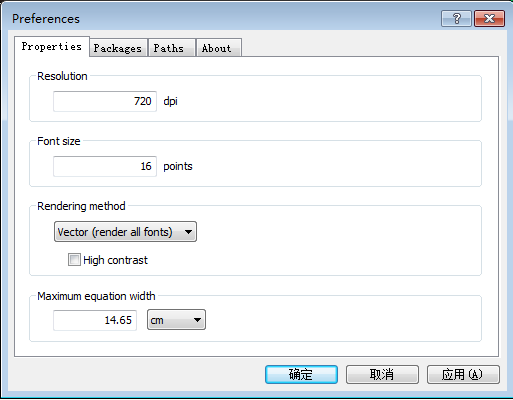Rendering method指的是渲染方式，一共有三种：Raster（位图），Vector（矢量图）和 Vector（render all fonts）。 如果有需要在没有安装Aurora插件的电脑的word查看公式，应使用第一种或第三种。但如果生成PDF再查看的话就不需要Aurora插件了。 miktex2.9使用第一种方式会报错 problem running latex，因此只能选择后两种。 具体的不同，可参见Aurora manual的Formula properties部分。

在编辑栏输入简单的latex代码后点击refresh进行测试，如果显示Done并在word文档的相应位置显示出编译得到的内容后，就证明安装成功。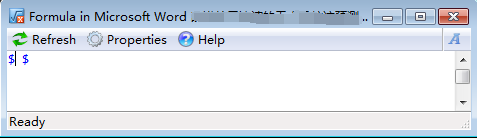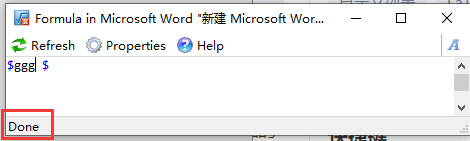以上内容全部在系统时间2009年的时候完成。如果显示Done之后，就可以把系统时间调回正常了。

2 编译遇到错误：Problems running LaTex
可能原因如下：
miktex的latex，dvipng和pdflatex路径不对；系统时间没有调整至2009年。在一次运行成功后就可以把时间调回来了；Rendering method 选择的不对。据说不同版本的miktex所能成功渲染的方法也不同，可以多试试； 修改了packages选项卡，引入了还没有安装的package（下文会讲）。
3 插入伪代码
首先，Aurora只是插件并无调试功能，任何在Aurora出现的问题都可以通过miktex调试，miktex成功了，Aurora就不会有大问题。因此，想要在Aurora中插入比较复杂的公式，需要引入一些包的时候，最好先在miktex中调试成功。使用miktex自带编辑器TeXworks就可以。   其次，由于之前我安装的是basic版的miktex，包含的宏包较少。如果直接在Aurora的packages选项卡引入没有安装过的宏包时，系统就会报错Problem running Latex。因此，需要提前在miktex中进行调试，因为此时系统会根据代码自动安装需要的宏包，例如，\usepackage{mathtools}这句话会让系统自动搜索并安装mathtools宏包。   如果想要进行包管理的话，可以打开miktex console的packages选项卡查看宏包的安装情况。在这里也可以进行宏包的安装和卸载。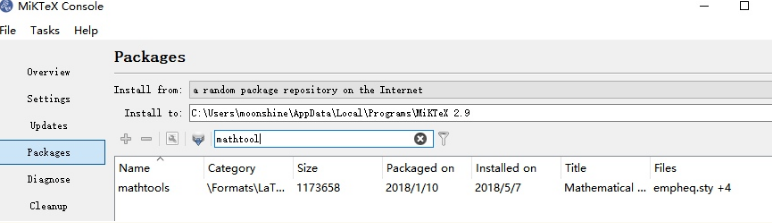4 举个栗子
假设以下宏包都已经在miktex中成功安装。在word中插入Aurora对象，并修改packages选项卡为以下内容：
\usepackage{amsmath}
\usepackage{amssymb}
% \usepackage{euler}
\providecommand{\abs}{\left\lvert#1\right\rvert}
\providecommand{\norm}{\left\lVert#1\right\rVert}
\usepackage{bbm}
\usepackage{CJK}
\usepackage{listings}
\usepackage{xcolor}
\usepackage{listings}
\usepackage{amsmath,bm,graphicx,multirow,bm,bbm,amssymb,psfrag,algorithm,subfigure,color,mdframed,wasysym,subeqnarray,multicol}

\usepackage{algorithm}
\usepackage{algpseudocode}
\usepackage{amsmath}
\renewcommand{\algorithmicrequire}{\textbf{Input:}}
\renewcommand{\algorithmicensure}{\textbf{Output:}}

在公式框中粘贴以下代码。如果你的伪代码段在miktex编译通过但是在Aurora会出问题，试一试像如下代码一样，在\begin{algorithm}后加上[H]。
\renewcommand{\thealgorithm}{1}
\begin{algorithm}[H]
\caption{*******************************************}
\label{ABCLFRS}
\begin{algorithmic}
\Require{S,$\lambda$,T,k}
\Ensure{$\mathbf{w}_{222}$}\\
\textbf{initialize}: Set $\mathbf{w}_1 = 0$
\For{$t = 1,2,...,T$}
\State Choose $A_t \subset[m]$
\EndFor
\end{algorithmic}
\end{algorithm}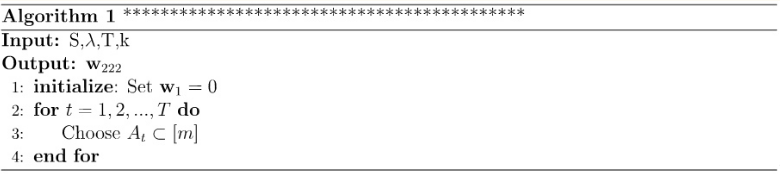展开全文• 实验内容: 对学生信息管理系统，要求完成以下基本任务： 1.改写程序为良好程序风格（文档注释，函数注释，语句注释）。 2.将功能补充完全（基于文件处理，完成...概要设计（包括数据结构及算法绘制流程图或伪代码表示）
实验内容:
对学生信息管理系统，要求完成以下基本任务：
1.改写程序为良好程序风格（文档注释，函数注释，语句注释）。
2.将功能补充完全（基于文件处理，完成刷新和保存功能）。
3.将学生信息改为更好的数据组织，而非离散形式（结构体）。
提高要求：
可将程序中的不足（如数据验证）等根据自己的能力与理解完成。
需求分析
1.1实现最基本的增删改查
1.2实现文件读入和文件保存功能
1.3利用结构体将学生信息改为更好的数据组织
1.4添加数据验证功能和文件刷新
概要设计（包括数据结构及算法绘制流程图或伪代码表示）
2.1数据结构
利用结构体存储学生信息
数组存储学生的三门课成绩
String存储学号，姓名，性别
2.2算法流程图
2.2.1整体设计流程图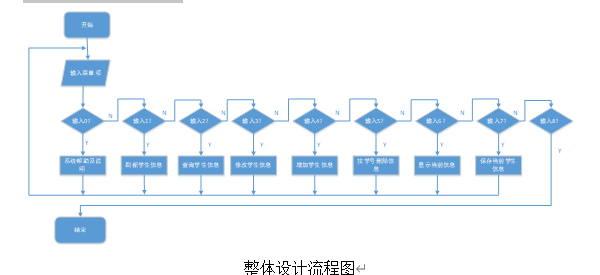2.2.2部分关键算法流程图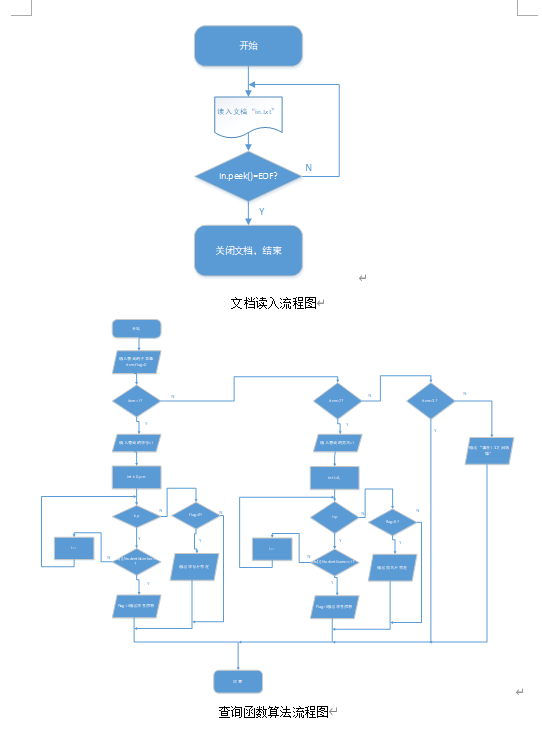源代码
下面展示一些 内联代码片。
// A code block
var foo = 'bar';



#include<stdio.h>      /*I/O函数*/

#include<stdlib.h>     /*其它说明*/

#include<string.h>    /*字符串函数*/

#include<iostream>

#include<fstream>

using namespace std;

#define LEN 15           /*
学号和姓名最大字符数,实际请更改*/

#define N 50       /*
最大学生人数,实际请更改*/

int k = 1, m = 0;/* n代表当前记录的学生人数*/

int n=0;

//函数声明

void seek();//查找学生信息

void modify();//修改学生信息

void insert();//增加学生信息

void del();//删除学生信息

void display();//显示当前信息

void save();//保存信息

//结构体保存学生信息

struct student{

string StudentId;//学号

string
StudentName;//姓名

int
StudentAge;//年龄

string
StudentSex;//性别

float
score;//成绩数组，分别记录C语言成绩，高等数学，学英语成绩

}stu[N];//结构体数组

//主函数

int main()

{

while (k)

{

}

return 0;

}

{

int i=0;

ifstream in("in.txt");//从"in.txt"文件中读入信息

while(in.peek()!=EOF)

{

i++;

printf("读入第%d位学生的学号:\n", i );

in>>stu[i].StudentId;

printf("读入第%d 个学生的姓名:\n",i);

in>>stu[i].StudentName;

printf("读入第%d 个学生的年龄:\n",i);

in>>stu[i].StudentAge;

printf("读入第%d 个学生的性别:\n",i);

in>>stu[i].StudentSex;

printf("读入第%d 个学生的C语言成绩\n",i);

in>>stu[i].score;

printf("读入第%d 个学生的高等数学成绩:\n",i);

in>>stu[i].score;

printf("读入第%d 个学生的大学英语成绩:\n",i);

in>>stu[i].score;

}

n=i+1;

in.close();//读入结束，关闭文件

}

//系统帮助函数

void help()

{

cout<<"\n0.欢迎使用系统帮助！"<<endl;

cout<<"\n1.初次进入系统后,请先选择增加学生信息;"<<endl;

cout<<"\n2.按照菜单提示键入数字代号;"<<endl;

cout<<"\n3.增加学生信息后,切记保存;"<<endl;

cout<<"\n4.谢谢您的使用！"<<endl;

}

//刷新文件

int flush(){

char filename[LEN
+ 1];//文件名

int i = 0;

cout<<"请输入要刷新学生信息的文件名："<<endl;

cin>>
filename;//输入文件名

int j = 0;

FILE * fp;

if ((fp =
fopen(filename, "r")) == NULL) return 0;

//从文件读取信息

while
(fscanf(fp, "%s %s %s %d %d %d %d ", &stu[j].StudentId,
&stu[j].StudentName, &stu[j].StudentAge, &stu[j].StudentSex,
&stu[j].score, &stu[j].score, &stu[j].score) == 7) {

j++;

}

n = j;

cout<<"刷新成功!!!"<<endl;

return n;

}

//查找学生信息

void seek() /*查找*/

{

int i,
item, flag;int p=n;

string s1;
/* 以姓名和学号最长长度+1为准*/

cout<<"------------------"<<endl;

cout<<"-----1.按学号查询-----"<<endl;

cout<<"-----2.按姓名查询-----"<<endl;

cout<<"-----3.退出本菜单-----"<<endl;

cout<<"------------------"<<endl;

while (1)

{

cout<<"请选择子菜单编号:";

cin>>item;

flag
= 0;

switch
(item)

{

case
1://按学号查询

{

cout<<"请输入要查询的学生的学号:"<<endl;

cin>>s1;

for
(i = 1; i<=p; i++)

if
(stu[i].StudentId==s1)//字符串比较，如果两个学号相等

{

flag
= 1;

//输出该学号的学生的信息

cout<<"学生学号\t学生姓名\t年龄\t性别\tC语言成绩\t高等数学\t大学英语成绩\n";

cout<<"--------------------------------------------------------------------\n"<<endl;

cout<<stu[i].StudentId<<"\t"<<stu[i].StudentName<<"\t"<<stu[i].StudentAge<<"\t"<<stu[i].StudentSex<<"\t"<<stu[i].score<<"\t"<<stu[i].score<<"\t"<<stu[i].score<<endl;

}

if
(0 == flag)//学号不存在时给出提示

cout<<"该学号不存在！"<<endl;break;}

case
2://按姓名查询

cout<<"请输入要查询的学生的姓名:"<<endl;

cin>>s1;

for
(i = 1; i<=p; i++)

if
(stu[i].StudentName==s1)

{

flag
= 1;

//输出该学号的学生的信息

cout<<"学生学号\t学生姓名\t年龄\t性别\tC语言成绩\t高等数学\t大学英语成绩"<<endl;

cout<<"--------------------------------------------------------------------"<<endl;

cout<<stu[i].StudentId<<"\t"<<stu[i].StudentName<<"\t"<<stu[i].StudentAge<<"\t"<<stu[i].StudentSex<<"\t"<<stu[i].score<<"\t"<<stu[i].score<<"\t"<<stu[i].score<<endl;

}break;

if
(0 == flag)//姓名不存在时给出提示

printf("该姓名不存在！\n");

case
3:return;

default:printf("请在1-3之间选择\n");

}

}

}

//修改学生信息

void modify() /*修改信息*/

{

int i,
item, num = -1;

int p=n;

string
s1,s2; /* 以姓名和学号最长长度+1为准*/

cout<<"请输入要要修改的学生的学号:"<<endl;

cin>>
s1;//输入要要修改的学生的学号

for (i =
1; i <=p; i++){

if
(stu[i].StudentId==s1){/*比较字符串是否相等*/

num
= i;

cout<<"------------------"<<endl;

cout<<"1.修改姓名"<<endl;

cout<<"2.修改年龄"<<endl;

cout<<"3.修改性别"<<endl;

cout<<"4.修改C语言成绩"<<endl;

cout<<"5.修改高等数学成绩"<<endl;

cout<<"6.修改大学英语成绩"<<endl;

cout<<"7.退出本菜单"<<endl;

cout<<"------------------"<<endl;

while
(1)

{

cout<<"请选择子菜单编号:";

cin>>item;//输入子菜单编号

switch
(item)

{

case
1:

cout<<"请输入新的姓名:"<<endl;

cin>>stu[num].StudentName;//输入的新的姓名

break;

case
2:

cout<<"请输入新的年龄:"<<endl;

cin>>stu[num].StudentAge;//输入新的年龄

break;

case
3:

cout<<"请输入新的性别:"<<endl;

cin>>stu[i].StudentSex
;//输入的新的性别

break;

case
4:

cout<<"请输入新的C语言成绩:"<<endl;

cin>>stu[num].score
;//输入的新的C语言成绩

break;

case
5:

cout<<"请输入新的高等数学成绩:"<<endl;//输入的新的高等数学成绩

cin>>stu[num].score;

break;

case
6:

cout<<"请输入新的大学英语成绩:"<<endl;//输入的新的大学英语成绩

cin>>stu[num].score;

break;

case
7:   return;

default:cout<<"请在1-7之间选择"<<endl;

}

}

cout<<"修改完毕！请及时保存！"<<endl;

}

else{

cout<<"没有该学生学号!!!"<<endl;

}

}

}

void sort()//按学号排序

{

int i, j,
k, *p, *q, s;

string
temp, ctemp;int itemp=0;

float
ftemp;

for (i =
1; i<=n - 1; i++)

{

for
(j = n - 1; j>i; j--)

if
(stu[j - 1].StudentId> stu[j].StudentId)//如果前一个学号比后一个学号大

{

//交换学号

temp=stu[j
- 1].StudentId;

stu[j -
1].StudentId=stu[j].StudentId;

stu[j].StudentId=temp;

//交换姓名

temp=stu[j
- 1].StudentName;

stu[j
- 1].StudentName=stu[j].StudentName;

stu[j].StudentName=
temp;

//交换性别

ctemp
= stu[j - 1].StudentSex;

stu[j
- 1].StudentSex = stu[j].StudentSex;

stu[j].StudentSex
= ctemp;

//交换年龄

itemp
= stu[j - 1].StudentAge ;

stu[j
- 1].StudentAge = stu[j].StudentAge;

stu[j].StudentAge
= itemp;

//交换三科成绩

for
(k = 0; k<3; k++)

{

ftemp
= stu[j - 1].score[k];

stu[j
- 1].score[k] = stu[j].score[k];

stu[j].score[k]
= ftemp;

}

}

}

}

//添加学生信息函数

void insert()

{

int i = n,
j, flag;

cout<<"请输入待增加的学生数:"<<endl;

cin>>m;

if(m>0)

{

for(int
i=n-1;i<n+m;i++)

{

flag
= 1;

while
(flag)

{

flag = 0;

printf("请输入第%d位学生的学号:\n", i + 1);

cin>>
stu[i].StudentId;

for
(j = 0; j <= i; j++){

if
(stu[i].StudentId==stu[j].StudentId){//如果学号已经存在

cout<<"该学号已存在，请重新输入！"<<endl;

flag
= 1;

break;

}

}

}

printf("请输入第%d 个学生的姓名:\n",i+1);

cin>>stu[i].StudentName;

printf("请输入第%d 个学生的年龄:\n",i+1);

cin>>stu[i].StudentAge;

printf("请输入第%d 个学生的性别:\n",i+1);

cin>>stu[i].StudentSex;

printf("请输入第%d 个学生的C语言成绩\n",i+1);

cin>>stu[i].score;

if(stu[i].score<0||stu[i].score>100)//对输入的成绩进行判错，成绩应该在0-100之间

{

cout<<"输错了，请重新输入成绩" <<endl;

cin>>stu[i].score;

}

printf("请输入第%d 个学生的高等数学成绩:\n",i+1);

cin>>stu[i].score;

if(stu[i].score<0||stu[i].score>100)

{

cout<<"输错了，请重新输入成绩" <<endl;

cin>>stu[i].score;

}

printf("请输入第%d 个学生的大学英语成绩:\n",i+1);

cin>>stu[i].score;

if(stu[i].score<0||stu[i].score>100)

{

cout<<"输错了，请重新输入成绩" <<endl;

cin>>stu[i].score;

}

}

}

n
+= m;//学生数增加

cout<<"学生信息增加完毕！！！"<<endl;

}

//删除学生信息函数

void del()

{

int i, j,
flag = 0;

char
s1[LEN + 1];

printf("请输入要删除学生的学号:\n");

scanf("%s",
s1);

for (i =
0; i < n; i++){

if
(stu[i].StudentId==s1){

flag
= 1;

//要删除学生后面的学生往前移一位

for
(j = i; j <=n - 1; j++){

stu[j]
= stu[j + 1];//

}

}

}

//查找失败

if
(0 == flag){

printf("该学号不存在!!!\n");

}

if
(1 == flag){

printf("删除成功！！！");

//删除成功，学生人数减1

n--;

}

system("pause");

}

//显示全部数据信息

void display()

{

int i;

printf("学生学号 \t学生姓名 \t年龄   \t性别
\tC语言成绩\t高等数学 \t大学英语成绩\n");

printf("--------------------------------------------------------------------\n");

for
(i = 0; i<=n; i++)

{

cout<<stu[i].StudentId<<"\t"<<stu[i].StudentName<<"\t"<<stu[i].StudentAge<<"\t"<<stu[i].StudentSex<<"\t"<<stu[i].score<<"\t"<<stu[i].score<<"\t"<<stu[i].score<<endl;

}

}

void save(){

int i;

sort();

FILE *fp;

ofstream
out("in.txt");

for (i =
1; i < n; i++){

out<<stu[i].StudentId<<"\t"<<stu[i].StudentName<<"\t"<<stu[i].StudentAge<<"\t"<<stu[i].StudentSex<<"\t"<<stu[i].score<<"\t"<<stu[i].score<<"\t"<<stu[i].score<<endl;

}

printf("保存成功！！！\n");

out.close();

}

{

int num;

printf("
\n\n                    \n\n");

printf("
******************************************************\n\n");

printf("  *                学生信息管理系统                    *\n \n");

printf("
******************************************************\n\n");

printf("*********************系统功能菜单*************************       \n");

printf("     ----------------------   ----------------------   \n");

printf("
*********************************************     \n");

printf("     * 0.系统帮助及说明  * *  1.刷新学生信息   *     \n");

printf("
*********************************************     \n");

printf("     * 2.查询学生信息    * *  3.修改学生信息   *     \n");

printf("
*********************************************     \n");

printf("     * 4.增加学生信息    * *  5.按学号删除信息 *     \n");

printf("
*********************************************     \n");

printf("     * 6.显示当前信息    * *  7.保存当前学生信息*     \n");

printf("     **********************
**********************     \n");

printf("     * 8.退出系统        *                            \n");

printf("     **********************                            \n");

printf("     ----------------------   ----------------------                           \n");

printf("请选择菜单编号:");

scanf("%d",
&num);

switch (num)

{

case
0:help(); break;

case
1:flush(); break;

case
2:seek(); break;

case
3:modify(); break;

case
4:insert(); break;

case
5:del(); break;

case
6:display(); break;

case
7:save(); break;

case 8:

k =
0;

printf("即将退出程序!\n");

break;

default:printf("请在0-8之间选择\n");

}

}


展开全文c++
• 常用加密算法的Java实现总结 ——对称加密算法DES、3DES和AES 日期：2014/7/6 文：阿蜜果 1、对称加密算法 1.1 定义 对称加密算法是应用较早的加密算法，技术成熟。在对称加密算法中，数据发信方...
 常用加密算法的Java实现总结
——对称加密算法DES、3DES和AES
日期：2014/7/6
展开全文java 加密
• 内存管理算法优化及在游戏引擎中的实现An Optimized Memory Manager and Application to Game Engine( 1.上海大学通信与信息工程学院2.西安电子科技大学电子工程学院) 周政春1 吴楷2 万旺根1Zhou,Zhengchun Wu,Kai ...引擎 优化 microsoft
• 1、对称加密算法 1.1 定义 对称加密算法是应用较早的加密算法，技术成熟。在对称加密算法中，数据发信方将明文（原始数据）和加密密钥（mi yue）一起经过特殊加密算法处理后，使其变成复杂的加密密文发送...加密
•   在GC 中最重要的算法就是GC标记-清除算法(Mark-Sweep GC)。在很多的场景下都还是在使用这个算法来进行垃圾回收操作。 什么是GC标记-清除算法   GC标记清除是由两个阶段构成的，一个是标记阶段，就是把所有...链表 数据结构
• 分布式中有这么一个疑难问题，客户端向一个分布式集群的服务端...要确保数据一致，需要选举算法的支撑，这就引申出了今天我们要讨论的题目，关于选举算法的原理解释及实现，选举包括对机器的选举，也包括对消息的选举。
• Deep SORT是多目标跟踪(Multi-Object Tracking)中常用到的一种算法，是一个Detection Based Tracking的方法。...代码)对Deep SORT算法进行代码层面的解析。 在之前笔者写的一篇Deep SORT论文阅读总结中...人工智能 深度学习 python
• ## redis的LRU算法实现

千次阅读 2019-03-31 01:00:32
Redis中采用两种算法进行内存回收，引用计数算法以及LRU算法，在操作系统内存管理一节中，我们都学习过LRU算法(最近最久未使用算法),那么什么是LRU算法呢 LRU算法作为内存管理的一种有效算法,其含...redis
• 使用python实现RSA算法，先简单的对RSA进行说明： 在对称密码体制中，密钥分发过程复杂，代价高；密钥量增大造成密钥管理困难；保密通信系统的开放性差；如果收发双方素不相识，或没有可靠的密钥传递渠道，则无法...
• 1、对称加密算法 1.1 定义 对称加密算法是应用较早的加密算法，技术成熟。在对称加密算法中，数据发信方将明文（原始数据）和加密密钥（mi yue）一起经过特殊加密算法处理后，使其变成复杂的加密密文发送...
• ## 算法

千次阅读 2016-11-24 16:07:02
基本上，这文章可以说是最佳A*算法文档。极力推荐！ Amit's A star Page中译文   译序 这篇文章很适合A*算法的初学者，可惜网上没找到翻译版的。本着好东西不敢独享的想法，也为了锻炼一下英文，本人译了这篇文章。...
• 2.4.5 用伪代码表示算法 30 2.4.6 用计算机语言表示算法 31 2.5 结构化程序设计方法 31 3 数据类型、运算符与表达式 3.1 Ｃ语言的数据类型 32 3.2 常量与变量 33 23.2.1 常量和符号常量 33 3.2.2 变量 33 3.3 整型...
• ## 操作系统读者写者问题代码实现

千次阅读 多人点赞 2019-05-14 02:52:53
伪代码： 读者优先： 读者R： Wait(rmutex); rcount++; if(rcount == 1) Wait(wmutex); Signal(rmutex); Read_Action(); Wait(rmutex); rcount--; if(rcount == 0) Signal(wmutex); Signal(rmutex); 写者W...读者写者问题 互斥
• 1、对称加密算法 1.1 定义 对称加密算法是应用较早的加密算法，技术成熟。在对称加密算法中，数据发信方将明文（原始数据）和加密密钥（mi yue）一起经过特殊加密算法处理后，使其变成复杂的加密密文发送...
• 算法伪代码： 2. 解码及计算适应度  将优化目标定义为总加工时间最短，因此适应度定义为最短加工时间的倒数，设fitness为对应个体的适应度，fulfillTime为最短加工时间，因此  其中fulfillTime的...
• 2.4.5 用伪代码表示算法 30 2.4.6 用计算机语言表示算法 31 2.5 结构化程序设计方法 31 3 数据类型、运算符与表达式 3.1 Ｃ语言的数据类型 32 3.2 常量与变量 33 23.2.1 常量和符号常量 33 3.2.2 变量 33 3.3 整型...
• 我们相信选择一种描述语言，与和系统其它部分揉合在一块的显式代码实现相比，要更容易对状态机进行推导和修改。 运行时一致性检查 ：虽然算法正确，但是人为错误、代码bug会导致系统不一致， 所以工程上添加周期...paxos 一致性
• ## Deep-Sort多目标追踪算法代码解析

千次阅读 多人点赞 2020-05-07 13:21:46
Deep SORT是多目标跟踪(Multi-Object Tracking)中常用到的一种算法，是一个Detection Based Tracking的方法。这个算法工业界关注度非常高，在知乎上有很多文章都是使用了Deep SORT进行工程部署。 1. MOT主要步骤 在...
• CMS （内容管理系统） CMS是Content Management System的缩写，意为"内容管理系统"。 内容管理系统是企业信息化建设和电子政务的新宠，也是一个相对较新的市场。对于内容管理，业界还没有一个统一的定义，不同的...
• 原文出处:... 原文出处：MSDN Magazine November 2003 (Encrypt It) ...本文的代码下载：msdnmag200311AES.exe (143KB) 本文假设你熟悉 C# 和 位（bit）操作。 摘要 　AES（The AAES aes c# C#
• 2、减少文件读取操作，采取小文件加判断调用，智能装载所需代码，提高网页反应速度。 3、更新smarty至3.0.8正式版，速度更快更稳定。 4、删除语言包文件，输出语言直接写入对应模板中，不再单列。 5、系统配置文件...云计算
• ## C语言课设销售管理系统设计

万次阅读 多人点赞 2016-05-26 09:21:01
该设计要求学生以某公司销售管理业务为背景，设计、开发一套“销售管理系统”软件。 通过该题目的设计过程，可以培养学生结构化程序设计的思想，加深对高级语言基本语言要素和控制结构的理解，针对c语言中的重点和...C C++ c语言
• 文章目录1 引言1.1 编写目的1.2 项目背景1.3 定义1.4 参考资料2 总体设计2.1 需求概述2.2 ...以下叙述将结合文字描述、伪代码，图表等来描述高校图书管理系统的详细设计和相关的模块描述。本报告的预期读者有客户、项软件工程 详细设计
• 机器学习算法与Python实践这个系列主要是参考《机器学习实战》这本书。... 在这一节我们主要是对支持向量机进行系统的回顾，以及通过Python来实现。由于内容很多，所以这里分成三篇博文。第一篇讲SVM初
• A model for entity predicting，实体(entity)是描述一些数据对象区别于其他数据对象的内容，可以理解为一种类型、标签。本文将介绍一种简单可行的算法(medrank配合B+树)来实现一个简单的实体预测模型。entity 数据 标签...